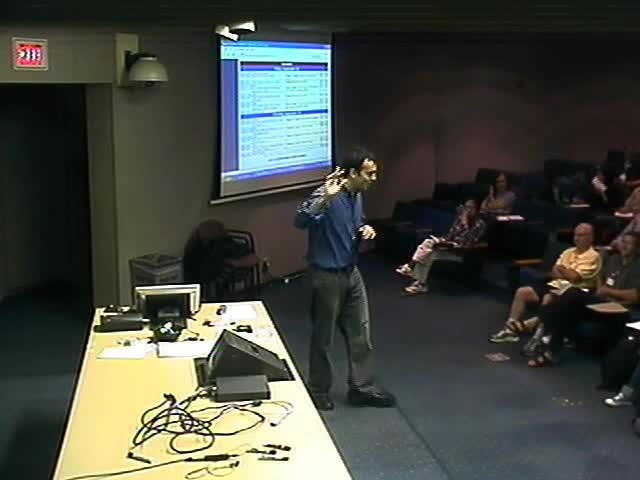### Videos

#### Mechanisms and Robot Kinematics: Algebraic Foundations

##### Presenter
September 16, 2006
Keywords:
• Mechanisms
MSC:
• 70B15##### Abstract
This talk will discuss how many of the basic questions in mechanism science are naturally formulated as systems of polynomial equations. These questions include both analysis problems (how does this mechanism move?) and synthesis problems (which mechanisms will move the way I require?). Once such a problem has been formulated as a system polynomials, the irreducible decomposition of the solution set of the system becomes a powerful tool for describing the answer. We will discuss how some questions can be approached in stages by computing irreducible decompositions of subsets of the original equations and then intersecting solution components. Finally, we will see how some questions about the existence of special mechanisms are nicely formulated as fiber products of algebraic sets.